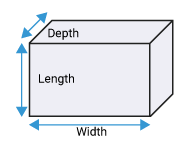Result:

## About Our Cubic Yard Calculator

### What is a Cubic Yard?

A cubic yard is a unit of volume used in the US. It is defined as the volume of cubes with the sides 1 yard each that is 36 inches. 1 cubic yard equals 27 cubic feet. A cubic yard is used to measure the three dimension volume or to find out how much that particular space can hold.

### How to Calculate Cubic Yards?

A cubic yard is calculated to fill a particular area with the material. To calculate cubic yards all you have to know is the length, width and depth. If you have these three then by using the formula you can get the cubic yard.

The formula for cubic yard is

length × width × height
27

If the units are not into feet then first we have to convert all the units into feet and then apply the Cubic yard formula.

### What is the Difference Between a Yard and a Cubic Yard?

A Cubic yard is a measurement used to measure a three-dimensional area of volume that is equal to a cube with 1 yard of length,1 yard of width and 1 yard of height. Whereas a yard is a unit used to measure two dimension area of measure equals 3 feet which are 36 inches.

### How to Use the Cubic Yard Calculator?

Cubic yard calculator is an online application that will calculate the cubic yard for you. All you have to do is enter the correct units of length in feet, width in feet and height in inches then after pressing enter you will get your answer.

### What Materials Are Measured in Cubic Yards?

A cubic yard is used to measure how much a particular space can hold a material. some of the common materials that are measured in a cubic yard are sand, concrete, gravel, dirt, topsoil, rock, compost, mulch etc.

### How Does the Cubic Yard Calculator Work?

A cubic yard calculator simply uses the formula that is length*width*height / 27. All you have to do is enter your length, weight and height in the spaces devoted to the particular units then the calculator will give you the answer in seconds.

### What Do You Need to Know Before Using the Cubic Yard Calculator?

A cubic yard calculator is used to calculate the volume of the cubic yard so that it becomes easy for you to know how much material that particular space is going to hold.

The formula for measuring the cubic yard is

length × width × height
27

So before using the formula you need to know the exact length, width and height of the particular space.

### How to Convert Cubic Feet to Cubic Yards?

As we have seen earlier there are 3 feet in 1 yard and 27 cubic feet in 1 cubic yard which means 3 feet * 3 feet * 3 feet. Firstly we need to convert the height which is into inches into feet then we have to multiply all the three dimensions of feet to get cubic feet then we have to divide that cubic foot by 27 to get the cubic yard. or we can simply use the Yardage Calculator to do the magic.

### How to Convert Cubic Inches to Cubic Yards?

To measure the cubic yard firstly you have to convert all the measurements into feet. now we know that 1 cubic yard is equal to 46656 cubic inches. that means in 1 cubic yard there are 36 inches * 36 inches * 36 inches. so to convert inches into yards you have to divide the given inches by 36. for example if you want to calculate a cubic yard with 24 feet width,24 feet length and 12-inch height then how much the given space can hold the concrete?

Firstly let us convert the inches into yards are 12inches / 36 inches = 0.33 yards,

Then let us convert feet to yards 34 feet / 3 = 8 yards

Then after multiplying all together we get 0.33 * 8 * 8 = 21.12 cubic yards.

Instead of doing these complex things manually, we can just put the figures in the cubic yard calculator and in seconds can get the result.

### How to Estimate a Volume From Square Feet to Cubic Yards?

Volume is the unit used to measure cubic yards that are yd3 and Square feet is the unit to measure the area that is ft2 . The formula for finding the area is length * width and the formula for finding volume is area * depth.

So if we multiply the area by the depth and height we get the volume in cubic feet then by dividing the volume by 27 we get the volume in a cubic yard. To cut this long process short you can just put the measures in the cubic yard calculator and in seconds your answer will get displayed.

A cubic yard is usually measured to find out how much material the given space can hold and to do so we can use the cubic yard calculator to make our work easier. Here are some Examples of Cubic yard calculator use.

1. A concrete cubic yard calculator can be used by the person who is working on a construction site as it will help him or her to know how much concrete that particular space can hold.
2. If you are planning to do gardening near your home then the cubic yard calculator can help you to find out how much area will be required for a particular space.
3. If you want to dispose of the garbage you can use this cubic yard calculator to find out the dumpster size so as to know how much debris will it hold.

History